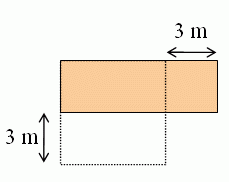### Shorter-wider table problem

I failed to publish this the other day since Blogger was acting up. I'll try it now.
A rectangular kitchen table is three times as long as it is wide.
If it were 3 m shorter and 3 m wider it would be a square.
What are the dimensions of the rectangular table?

1) You can solve this using algebra: set width to be x, the length is then 3x. We know these two will be equal if the former is increased by 3, and the latter is decreased by 3:
x + 3 = 3x - 3

2x = 6

x = 3.
The dimensions are 3 and 9.

But since this is supposed to be a 6th grade problem, surely we can find another way to solve it, as well.

2) Think of the two quantities length and width as just numbers. If you reduce one by 3, and increase the other by 3, they will "meet" or be the same. Below, I've drawn the two numbers as lines; you could use bars.

length |---------------------------------|

width |----------|

If I decrease the length by 3 and increase the width by 3, they'll be equal:

length |---------------------|<------------|

width |----------|--------->|

So... the DIFFERENCE between these two is 6. The difference of 6 is also TWO TIMES the original width, because remember the length is 3 times the width.

So the original width is 3 m and the original length is 9 m (a giant table!).

3) Solving it geometrically is also quite easy. First draw a table that looks about 3 times as long as it is wide. Then trace the sides if it was a square, making the long side shorter, and short side longer:

It sure looks like that the side of the square should be double 3, or 6. The original sides would then be 3 and 9. And you can easily check out that those work.--------------------------------------------------------------

I feel like the numbers in this problem are almost too easy. But maybe this problem could be used as a stepping stone for a harder version of the same.